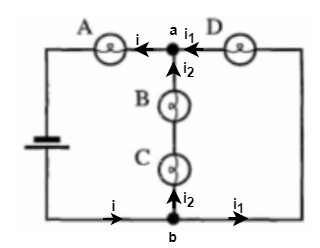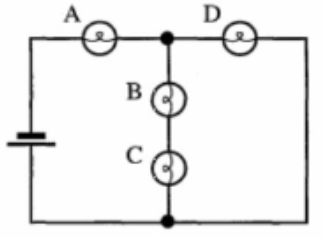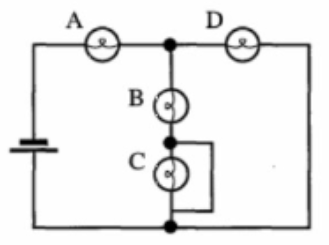# Problem: The circuit shown has four identical light bulbs and an ideal batterya. Rank the brightness of the bulbs. Explain your reasoning.b. A wire is now added to the circuit as shown.i. Does the brightness of bulb C increase, decrease or remain the same? Explain your reasoning.ii. Does the brightness of bulb A increase, decrease, or remain the same? Explain.iii. Does the current through the battery increase, decrease, or the remain the same? Explain.

###### FREE Expert Solution

a.

Since all the bulbs are identical, we take the resistance of each bulb to be R.

Power is expressed as:

$\overline{){\mathbf{P}}{\mathbf{=}}{{\mathbf{i}}}^{{\mathbf{2}}}{\mathbf{R}}}$

From this equation, the current is directly proportional to power.

Ohm's law:

$\overline{){\mathbit{i}}{\mathbf{=}}\frac{\mathbf{V}}{\mathbf{R}}}$

We need to determine the current flowing in each branch.

Let's re-label the figure as below.The currents are drawn based on Kirchhoff's junction rule.

95% (293 ratings)###### Problem Details

The circuit shown has four identical light bulbs and an ideal battery
a. Rank the brightness of the bulbs. Explain your reasoning.b. A wire is now added to the circuit as shown.i. Does the brightness of bulb C increase, decrease or remain the same? Explain your reasoning.ii. Does the brightness of bulb A increase, decrease, or remain the same? Explain.
iii. Does the current through the battery increase, decrease, or the remain the same? Explain.

Frequently Asked Questions

What scientific concept do you need to know in order to solve this problem?

Our tutors have indicated that to solve this problem you will need to apply the Power in Circuits concept. You can view video lessons to learn Power in Circuits. Or if you need more Power in Circuits practice, you can also practice Power in Circuits practice problems.

What professor is this problem relevant for?

Based on our data, we think this problem is relevant for Professor Castano's class at NSU.